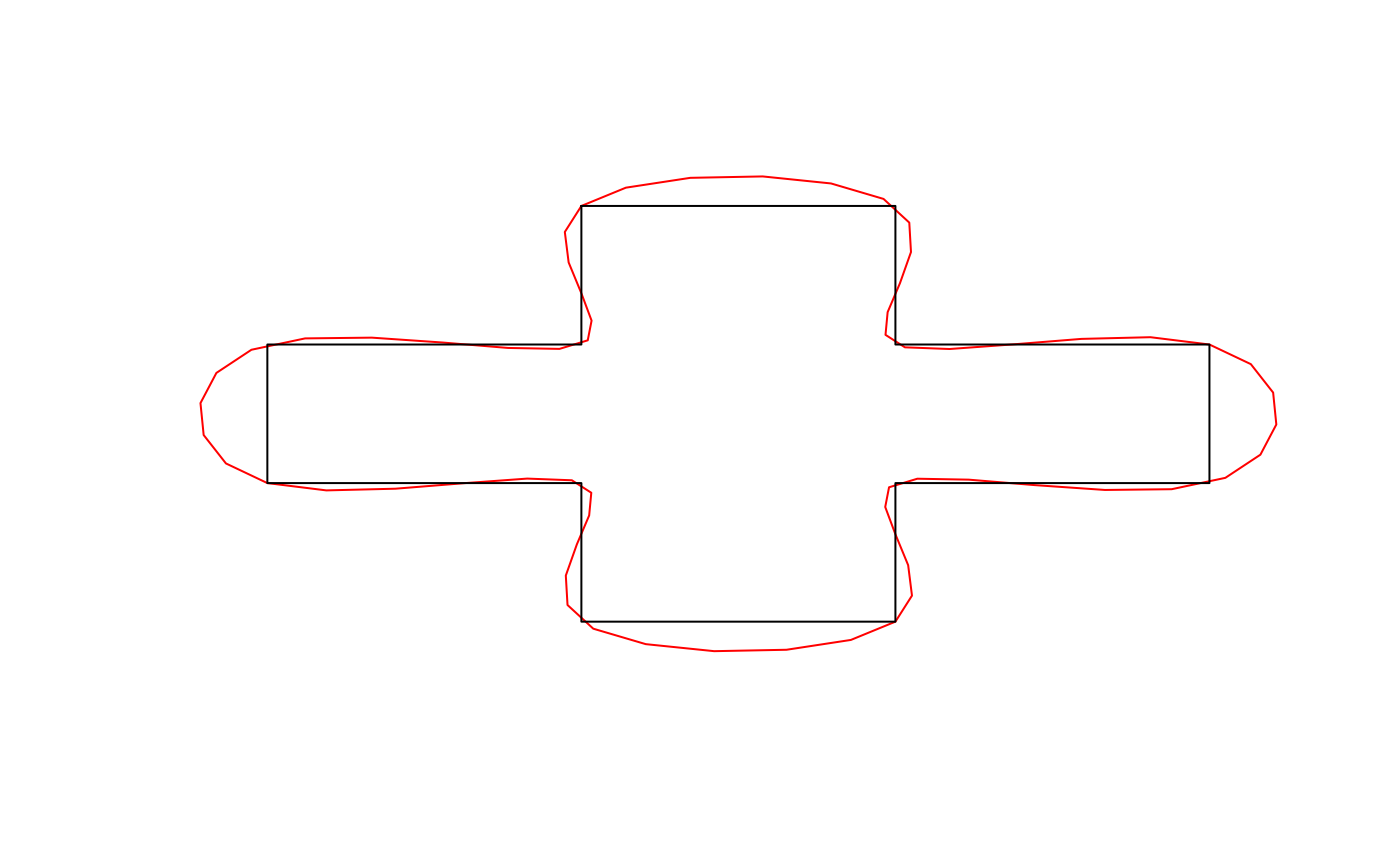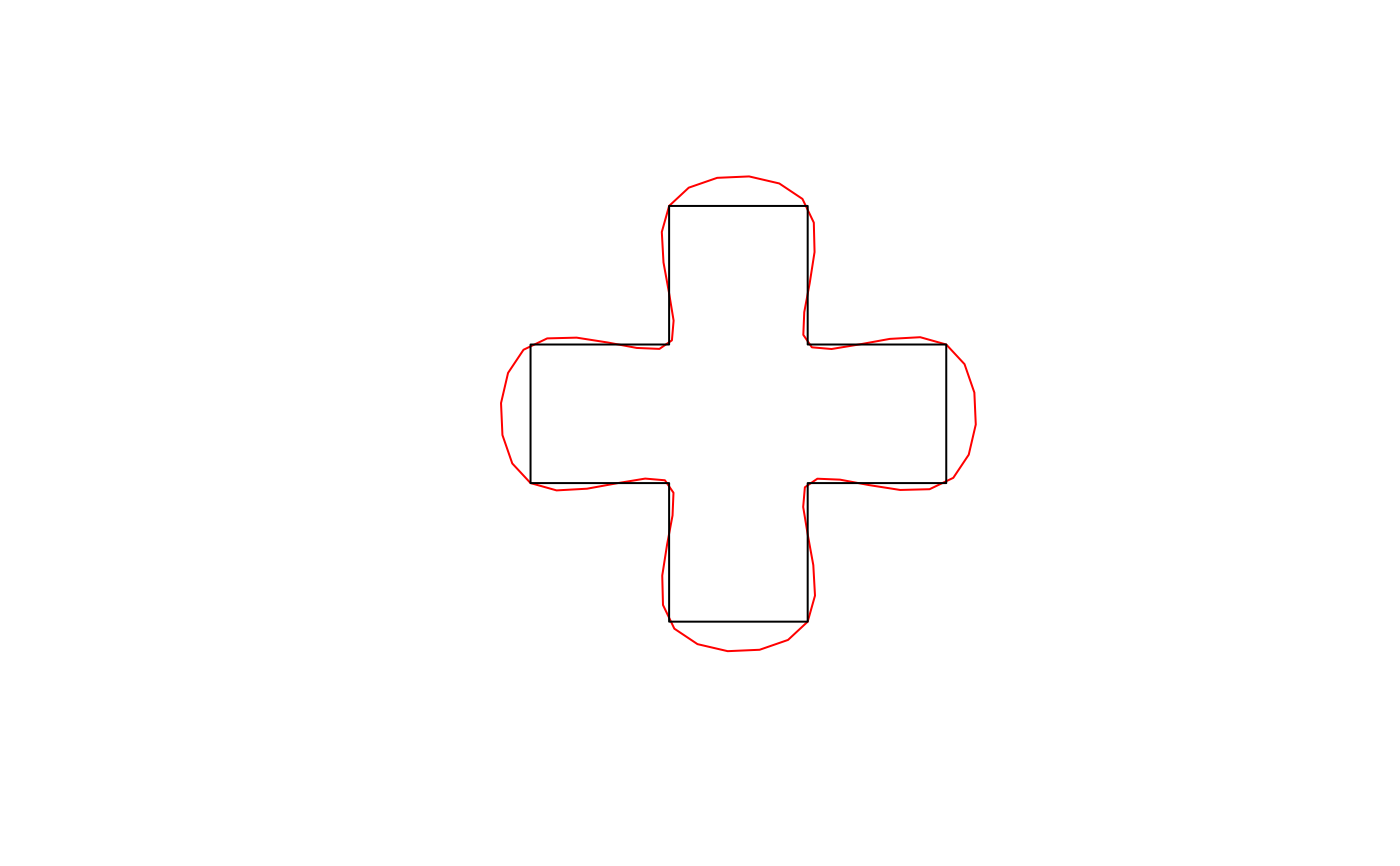Spline interpolation uses stats::spline() to interpolate between existing vertices using piecewise cubic polynomials. The coordinates are interpolated independently. The curve will always pass through the vertices of the original feature.

smooth_spline(x, wrap = FALSE, vertex_factor = 5, n)

## Arguments

x numeric matrix; matrix of coordinates. logical; whether the coordinates should be wrapped at the ends, as for polygons and closed lines, to ensure a smooth edge. double; the proportional increase in the number of vertices in the smooth curve. For example, if the original curve has 100 points, a value of 2.5 will yield a new smoothed curve with 250 points. Ignored if n is specified. integer; number of vertices in the smoothed curve.

## Value

A matrix with the coordinates of the smoothed curve.

## Details

This function works on matrices of points and is generally not called directly. Instead, use smooth() with method = "spline" to apply this smoothing algorithm to spatial features.

The spline method was inspired by the following StackExchange answers:

smooth()

## Examples

# smooth_spline works on matrices of coordinates
# use the matrix of coordinates defining a polygon as an example
m <- jagged_polygons$geometry[][] m_smooth <- smooth_spline(m, wrap = TRUE) class(m) #>  "matrix" "array" class(m_smooth) #>  "matrix" "array" plot(m_smooth, type = "l", col = "red", axes = FALSE, xlab = NA, ylab = NA) lines(m, col = "black")# smooth is a wrapper for smooth_spline that works on spatial features library(sf) p <- jagged_polygons$geometry[]
p_smooth <- smooth(p, method = "spline")
class(p)
#>  "XY"      "POLYGON" "sfg"    class(p_smooth)
#>  "XY"      "POLYGON" "sfg"    plot(p_smooth, border = "red")